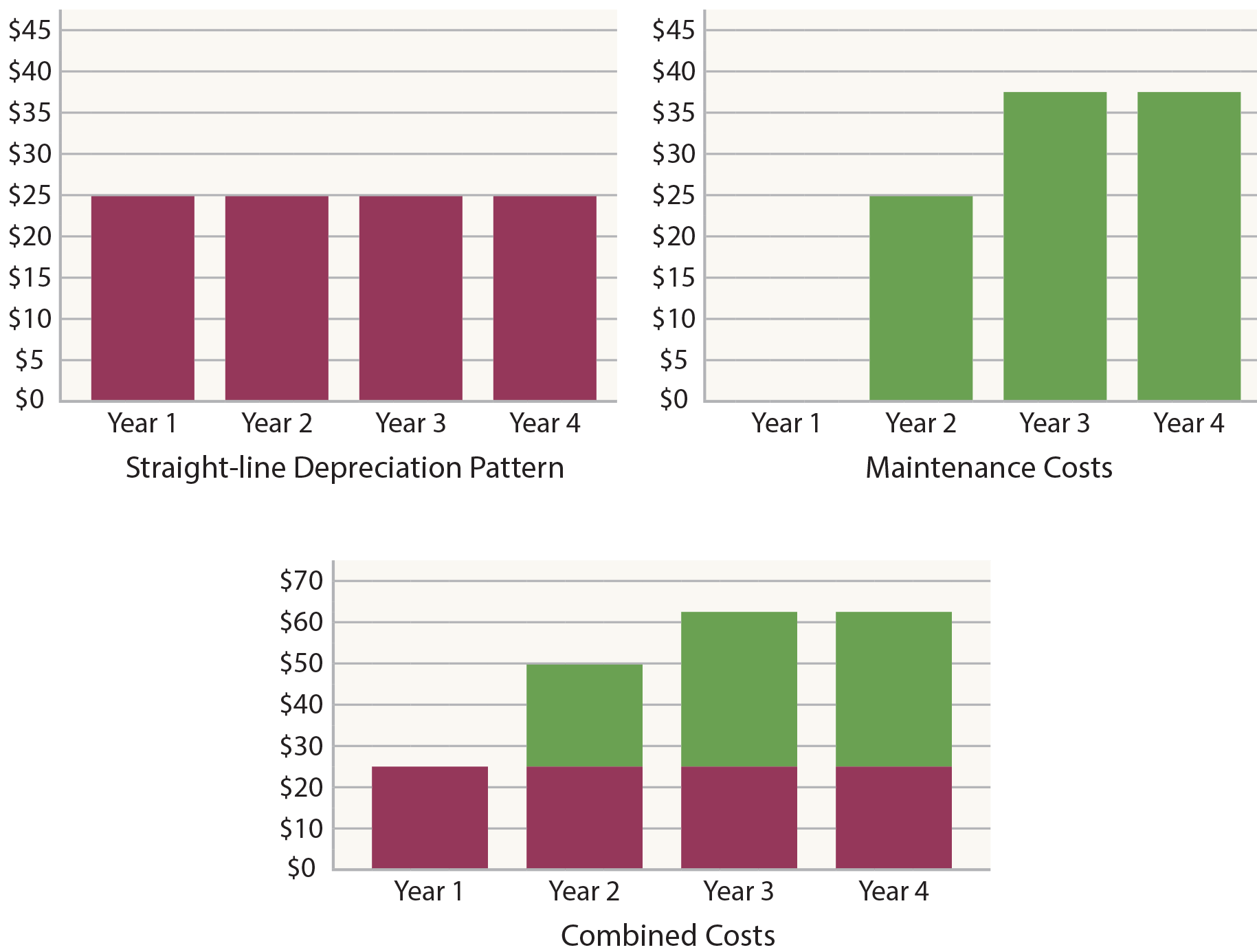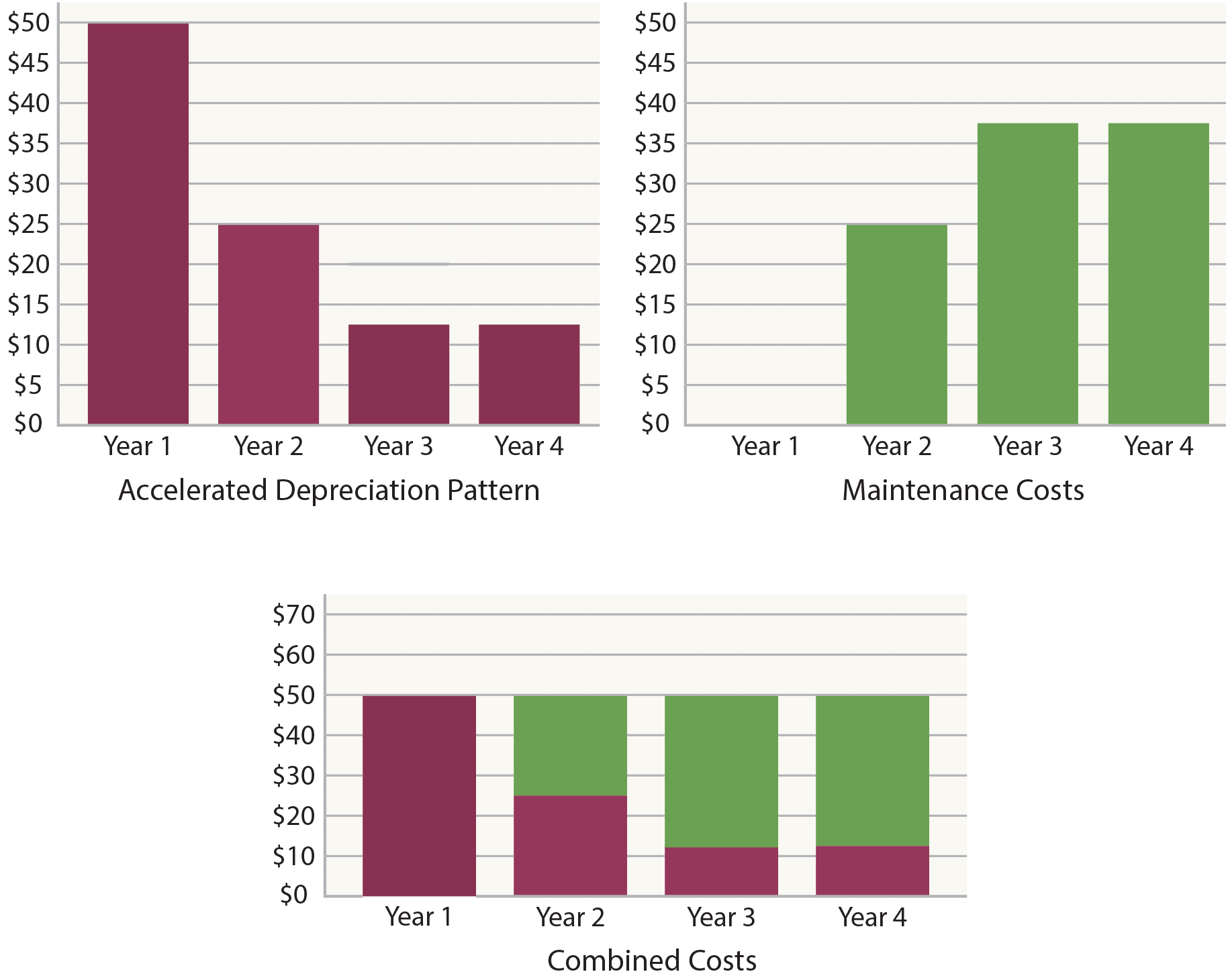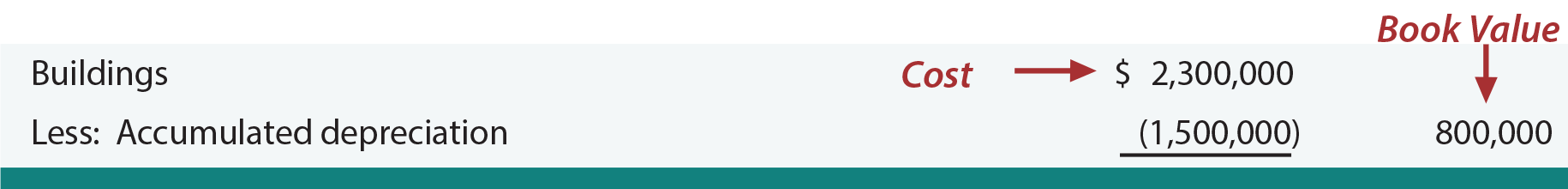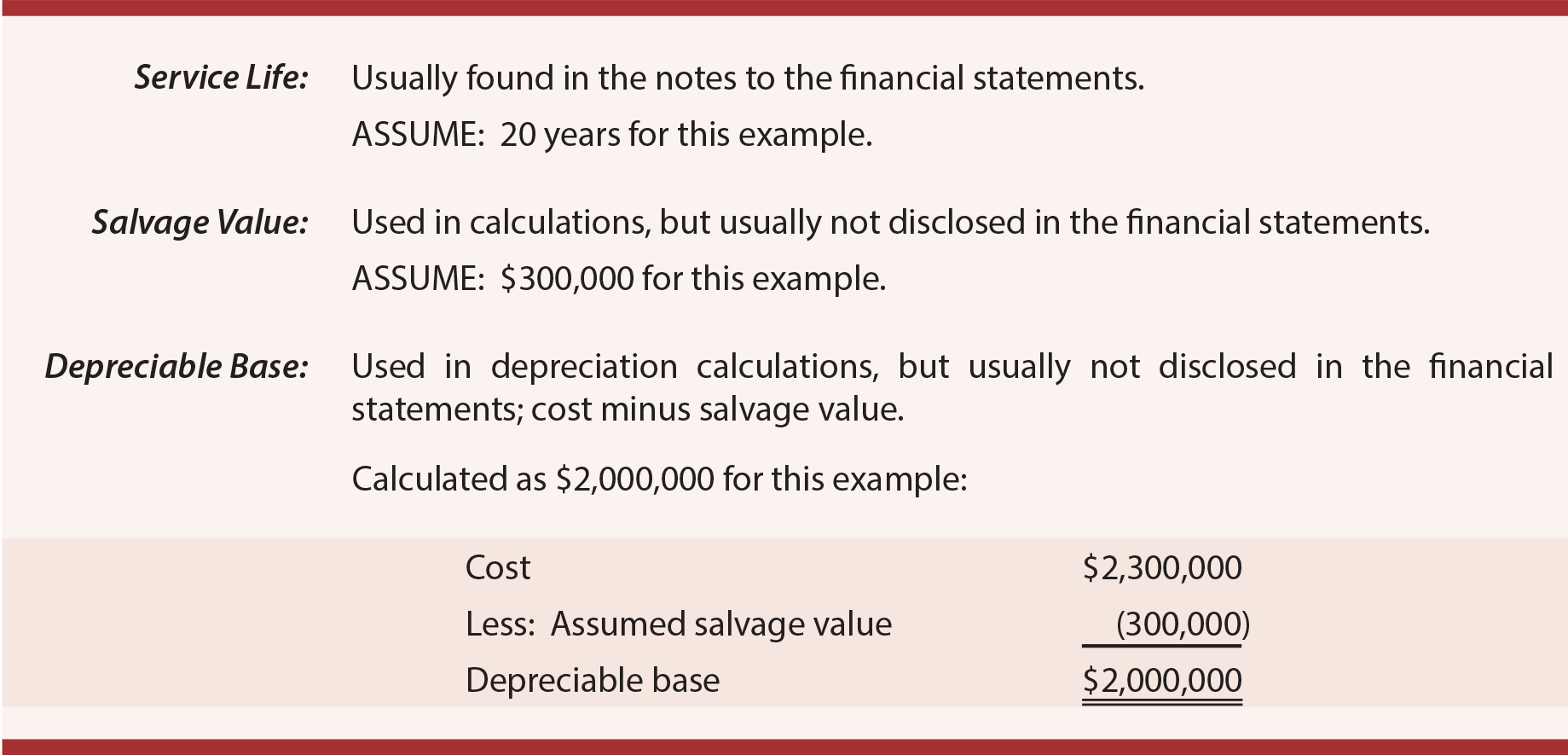Once the cost and service life of an asset are determined, it is time to move on to the choice of depreciation method. The depreciation method is simply the pattern by which the cost is allocated to each of the periods involved in the service life. There are many methods from which to choose. Three popular methods are:

• straight-line
• units-of-output, and
• double-declining balance

Why so many choices? To explain, begin by assuming that a \$100 asset is to be depreciated over four years. Under the straight-line approach, depreciation expense is simply \$25 per year (shown in burgundy below). This may seem very logical if the asset is used uniformly over the four-year period. But, what if maintenance costs (shown in green) are also considered? As an asset ages, it is not uncommon for maintenance costs to expand. Assume the first-year maintenance is \$0 and rises each year as shown. Combining the two costs together reveals an increase in total cost, even though the usage is deemed to be constant.

With accelerated depreciation (shown below), the combined amount of depreciation and maintenance provides a level measure of total cost. This may achieve a better matching of total costs and benefits in this particular scenario. Does this mean that accelerated depreciation is better? Not necessarily. Facts can vary, and the point is to show why multiple methods exist and can be justified.

#### Straight-Line Effects#### Accelerated Effects### Some Important Terminology

In any discipline, precision is enhanced by adopting terminology that has very specific meaning. Accounting for Property, Plant, & Equipment is no exception. An exact understanding of the following terms is paramount:

• Cost: The dollar amount assigned to a particular asset, usually the ordinary and necessary amount expended to get an asset in place and in condition for its intended use.
• Service life: The useful life of an asset to an enterprise, usually relating to the anticipated period of productive use of the item.
• Salvage value: Also called residual value. This is the amount expected to be realized at the end of an asset’s service life; for example, the anticipated future sales proceeds for used equipment.
• Depreciable base: The cost minus the salvage value. Depreciable base is the amount of cost that will be allocated to the service life.
• Book value: Also called net book value. This refers to the balance sheet amount at a point in time that reveals the cost minus the amount of accumulated depreciation (book value has other meanings when used in other contexts, so this definition is limited to its use in the context of PP&E).

Below is an illustration relating these terms to the financial statement presentation for a building:In the preceding illustration, assuming straight-line depreciation, what is the asset’s age? The \$2,000,0000 depreciable base (\$2,300,000 – \$300,000) is evenly spread over 20 years. This produces annual depreciation of \$100,000. As a result, the accumulated depreciation of \$1,500,000 suggests an age of 15 years (15 X \$100,000).

Did you learn?
Be able to explain why multiple depreciation methods exist.
Define cost, service life, salvage value, depreciable base, and book value.